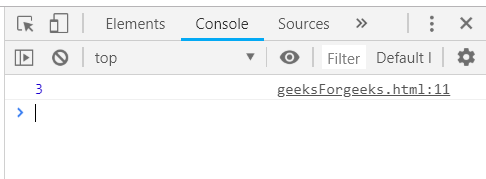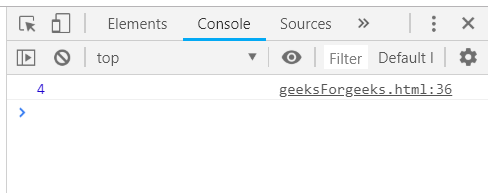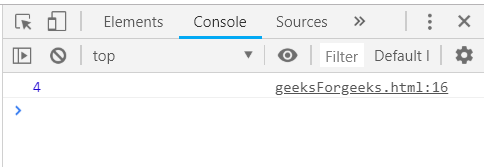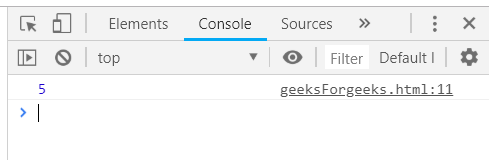# Underscore.js | _.size() with Examples

The Underscore.js is a JavaScript library that provides a lot of useful functions that helps in the programming in a big way like the map, filter, invoke etc even without using any built-in objects.
The _.size() function is used to find the size of an array, i.e. the number of elements in the array. It is mostly used to find the number of elements in an array.

Syntax:

`_.size( list ) `

Parameters: This function accepts single parameter list which is used to hold the list of items.

Return values: This function returns a value which is the size of the passed array.

Passing an array to the _.size() function: The ._size() function takes the element from the list one by one and calculate the size by starting from zero and increment by one as it passes through each element. Passing an array which has first the property name and then the element as one element of the array. If property name will not mentioned then the _.size() function will not work.

Example:

 ` ` `<``html``> ` `    ``<``head``> ` `        ``<``script` `src` `=  ` `"https://cdnjs.cloudflare.com/ajax/libs/underscore.js/1.9.1/underscore-min.js"` `> ` `        `` ` `    `` ` `    ``<``body``> ` `        ``<``script` `type``=``"text/javascript"``> ` `            ``var list = [1, 2, 3, 4, 5]; ` `            ``console.log(_.size(list)); ` `        `` ` `    `` ` `                     `

Output:Passing an array of large number of property to _.size() function: Passing a large array to the _.size() function. The _.size() function takes an array which has 4 properties, ‘category’, ‘title’, ‘value’ and ‘id’. Then apply _.size() function on this array and console.log() will display the final result.

Example:

 ` ` `<``html``> ` `    ``<``head``> ` `        ``<``script` `src` `=  ` `"https://cdnjs.cloudflare.com/ajax/libs/underscore.js/1.9.1/underscore-min.js"` `> ` `        `` ` `    `` ` `    ``<``body``> ` `        ``<``script` `type``=``"text/javascript"``> ` `            ``var goal = [ ` `            ``{ ` `                ``"category" : "other", ` `                ``"title" : "harry University", ` `                ``"value" : 50000, ` `                ``"id":"1" ` `            ``}, ` `            ``{ ` `                ``"category" : "traveling", ` `                ``"title" : "tommy University", ` `                ``"value" : 50000, ` `                ``"id":"2" ` `            ``}, ` `            ``{ ` `                ``"category" : "education", ` `                ``"title" : "jerry University", ` `                ``"value" : 50000, ` `                ``"id":"3" ` `            ``}, ` `            ``{  ` `                ``"category" : "business", ` `                ``"title" : "Charlie University", ` `                ``"value" : 50000, ` `                ``"id":"4" ` `            ``} ` `        ``] ` `        ``console.log(_.size(goal)); ` `        `` ` `    `` ` `                     `

Output:Passing a structure to the _.size() function: First declare an array (here array is ‘people’). Then just simply pass this array to the _.size() function and console.log() will display the final answer.

Example:

 ` ` `<``html``> ` `    ``<``head``> ` `        ``<``script` `src` `=  ` `"https://cdnjs.cloudflare.com/ajax/libs/underscore.js/1.9.1/underscore-min.js"` `> ` `        `` ` `    `` ` `    ``<``body``> ` `        ``<``script` `type``=``"text/javascript"``> ` `            ``var people = [ ` `            ``{"name": "sakshi", "hasLong": "false"}, ` `            ``{"name": "aishwarya", "hasLong": "true"}, ` `            ``{"name": "akansha", "hasLong": "true"}, ` `            ``{"name": "preeti", "hasLong": "true"} ` `        ``] ` `        ``console.log(_.size(people)); ` `        `` ` `    `` ` `                     `

Output:Passing only one property array to the _.size() function: Declare an array which contains one property. The array ‘users’ is defined with one property ‘num’. Then simply pass this array to _.size() function by mentioning the array’s name inside the _.size() function. Finally, console.log() will display the final answer.

Example:

 ` ` `<``html``> ` `    ``<``head``> ` `        ``<``script` `src` `=  ` `"https://cdnjs.cloudflare.com/ajax/libs/underscore.js/1.9.1/underscore-min.js"` `> ` `        `` ` `    `` ` `    ``<``body``> ` `        ``<``script` `type``=``"text/javascript"``> ` `            ``var users = [{"num":"1"}, ` `                         ``{"num":"2"},  ` `                         ``{"num":"3"},  ` `                         ``{"num":"4"},  ` `                         ``{"num":"5"} ` `        ``]; ` `        ``console.log(_.size(users)); ` `        `` ` `    `` ` `                     `

Output:My Personal Notes arrow_drop_upCheck out this Author's contributed articles.

If you like GeeksforGeeks and would like to contribute, you can also write an article using contribute.geeksforgeeks.org or mail your article to contribute@geeksforgeeks.org. See your article appearing on the GeeksforGeeks main page and help other Geeks.

Please Improve this article if you find anything incorrect by clicking on the "Improve Article" button below.

Article Tags :

Be the First to upvote.

Please write to us at contribute@geeksforgeeks.org to report any issue with the above content.